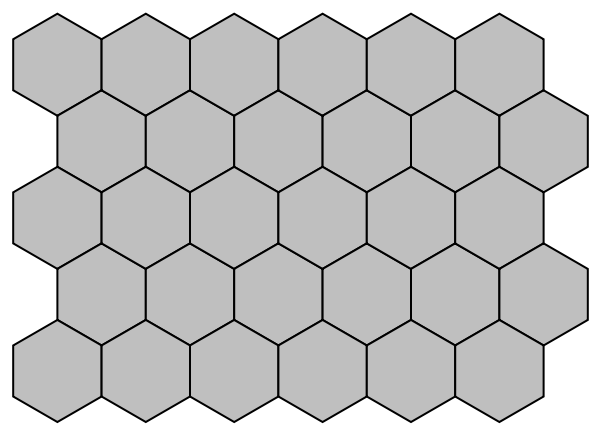#Function Repository Resource:

# TessellationPlot

Generate a tessellation of the plane with specified cell shapes

Contributed by: Jack Heimrath  |  Jack Heimrath
 TesselationPlot[array] generates a plot consisting of m⨯n square cells, where m and n are the dimensions of array, and the value array[[i,j]] specifies the content of the (i,j) cell. ResourceFunction["TessellationPlot"][array,basis] generates the tesselation plot induced by the lattice generated by the given basis vectors.

## Details and Options

ResourceFunction["TessellationPlot"] requires a real 2D array as input. This can take the form of a List or SparseArray.
The value of basis should be a basis of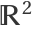.
The elements of array are used to determine the GrayLevel of the corresponding cell. As such, these values should be numeric. In simulating cellular automata, the array entries are usually restricted to {0,1}.
The cells generated by ResourceFunction["TessellationPlot"] are indexed in the same way as the array entries (left to right, top to bottom).
The following options can be given:
 "CellShape" "Square" shape of the cell ImageSize 300 the absolute size at which to render the graphic
The "CellShape" option allows types "Square", "Hexagon" and "Triangle".

## Examples

### Basic Examples (3)

Generate a regular 56 grid from a constant array:

 In:=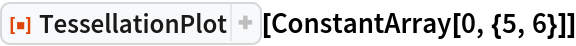Out=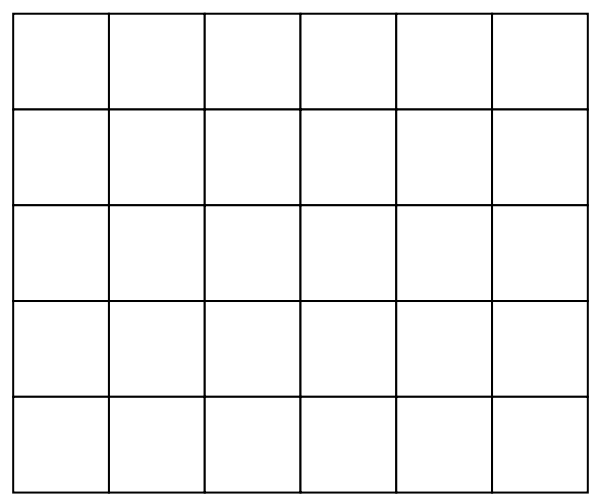Generate a regular 56 grid using random real values:

 In:=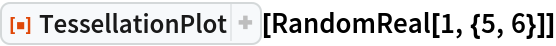Out=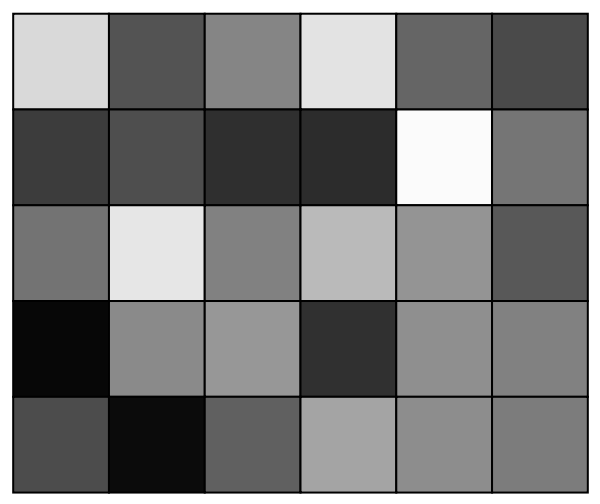Use a different basis for the lattice:

 In:=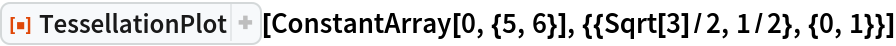Out=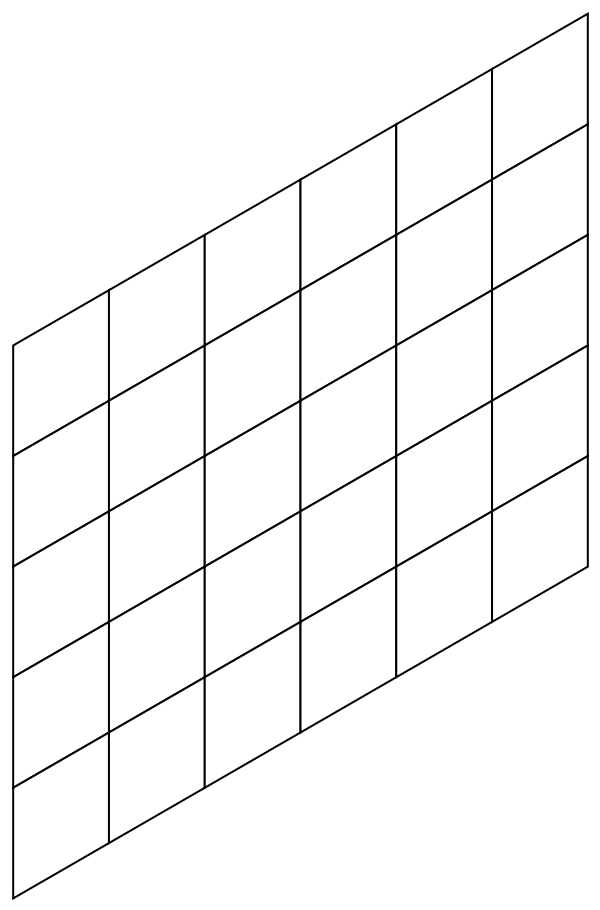### Options (2)

#### CellShape (2)

By default, TessellationPlot uses cells shaped like parallelograms:

 In:=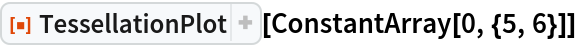Out=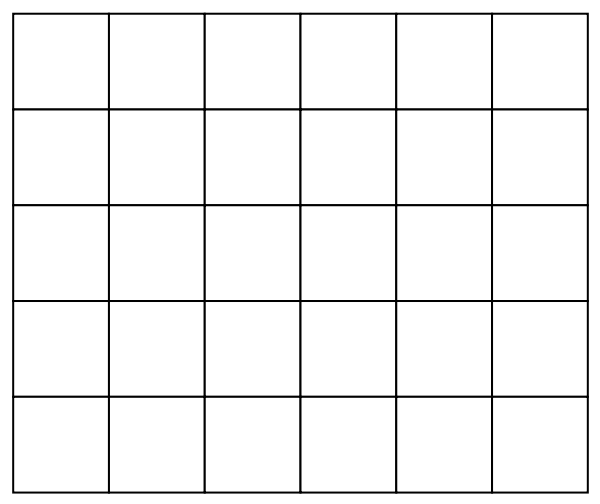Set "CellShape" to obtain different cells:

 In:=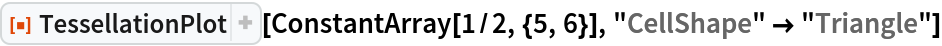Out=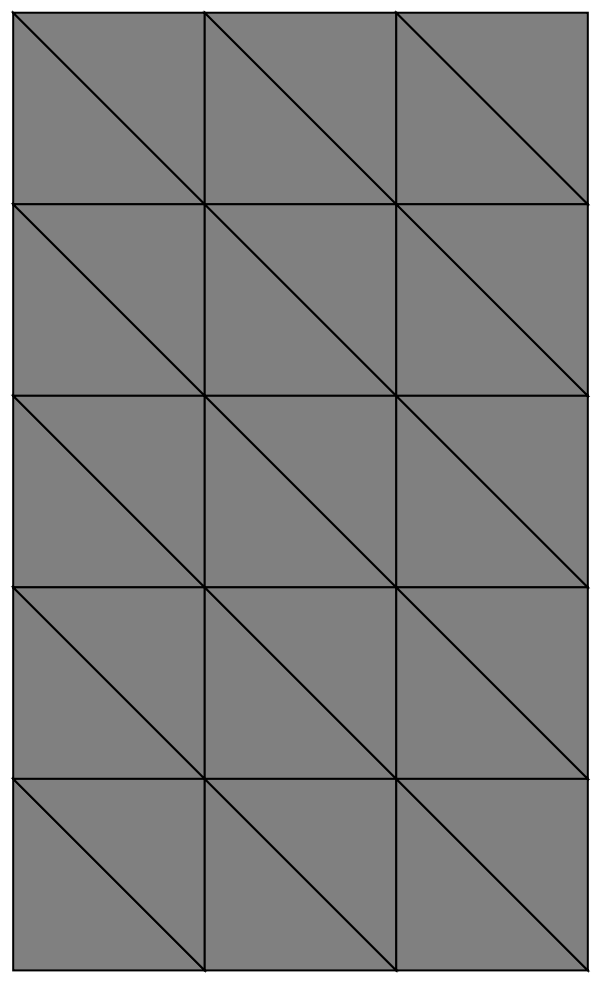In:=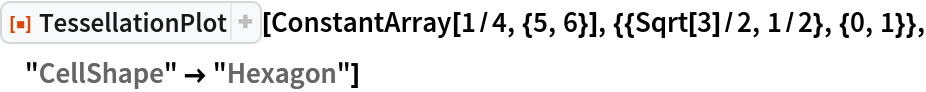Out=